## Thursday, April 28, 2016

### Multi View Stereo 10 (MVS10)

MVS10 builds a dense reconstruction of a 3D scene from a set of pictures (taken with a camera in photo mode or extracted from a video). Its input is a nvm file which comes either from Structure from Motion 10 (SfM10) or VisualSFM : A Visual Structure from Motion System and the images. The nvm file contains the camera poses (location and orientation) as well as a sparse 3D reconstruction of the scene. MVS10 is loosely based upon "Bundled Depth-Map Merging for Multi-View Stereo" by Jianguo Li, Eric Li, Yurong Chen, Lin Xu, Yimin Zhang. MVS10 pairs selected images/cameras, computes the corresponding disparity maps, and merges them as best as possible.

MVS10's workflow is as follows:
- Initialize the dense with 3D reconstruction with the sparse 3D reconstruction contained in the nvm file
- For each pair of images/cameras
-- Rectify the images using code similar to Epipolar Rectification 9b (ER9b)
-- Compute the disparity map using code similar to Depth Map Automatic Generator 5 (DMAG5)
-- Add the matches (pairs of image points) coming from the disparity map to the 3D reconstruction
-- Remove image points for which the reprojection error is too large
- Remove 3D points (also known as tracks in the literature) for which the number of image points is too low

The output of MVS10 is:
- A set of frames named duh_XX.jpg where XX varies from 01 to 11 that can be used to create an animated gif of the reconstructed 3D scene.
- A point cloud in ply format names duh.ply that describes the 3D scene which can be loaded and possibly meshed (I recommend using the Poisson method) in MeshLab.

MVS10 requires the presence of an input file called "mvs10_input.txt" containing the following parameters:
- Filename for nvm file. The nvm file is obtained from SfM10 or VisualSFM. The images referenced by the nvm file must of course also be present in the directory where MVS10 is run from.
- Number of trials used in rectification. The higher the number, the more accurate the rectification is and the more accurate the (good) matches are.
- Minimum average separation angle (camera pair selection). The separation angle for a given 3D point and a pair of images/cameras is the angle between the rays that go from the 3D point to the camera centers. If the average separation angle is too small, the disparity map is likely to be poor because there's not enough parallax between the two rectified images. If the average separation angle is below the given threshold, the disparity map is not computed and will obviously not participate in the 3D reconstruction.
- Radius used to smooth the cost. This parameter is used to compute the disparity map. It is explained in Depth Map Automatic Generator 5 (DMAG5).
- Alpha. This parameter is used to compute the disparity map. It is explained in Depth Map Automatic Generator 5 (DMAG5).
- Truncation value for color cost. This parameter is used to compute the disparity map. It is explained in Depth Map Automatic Generator 5 (DMAG5).
- Truncation value for gradient cost. This parameter is used to compute the disparity map. It is explained in Depth Map Automatic Generator 5 (DMAG5).
- Epsilon. This parameter is used to compute the disparity map. It is explained in Depth Map Automatic Generator 5 (DMAG5).
- Disparity tolerance used to detect occlusions. This parameter is used to compute the disparity map. It is explained in Depth Map Automatic Generator 5 (DMAG5).
- Downsampling factor. Because computing disparity maps takes time, downsampling images, that is reducing the size of images, is quite attractive because it can considerably reduce the computation time. At the expense of accuracy, of course. If the images are in the one megapixel range, downsampling is probably not needed but beyond that, downsampling by a factor of two or more can be much beneficial in terms of computation time.
- Sampling step. Each time a disparity map is computed, each and every pixel in one image can be matched to a pixel in the other image. Well, that can be very taxing computationally wise. If the sampling step is set to two, every other pixel is considered. If the sampling step is set to three, one out of every three pixels is considered. The less sampling, the more dense the 3D reconstruction is going to be.
- Minimum separation angle. This parameter is used to remove 3D points (aka tracks) that are low-confidence. If the separation angle is too low (below the given threshold), the 3D point is probably not accurate and is discarded. The lower the number, the more 3D points will remain in the 3D reconstruction and the denser it will be.
- Minimum number of image points per 3D point. This parameter is used to remove 3D points (aka tracks) that are low-confidence. The lower the number, the more 3D points will remain in the 3D reconstruction and the denser it will be.
- Maximum reprojection error. This parameter is used to remove for a given 3D point the image points that are low-confidence. The lower the reprojection error threshold, the more image points are gonna be discarded and the more 3D points will be discarded as a result.
- Radius for the animated gif frames. Instead of using a single pixel for each 3D point when computing the frames, a square is used whose dimensions are two times the radius plus one. The denser the 3D reconstruction, the lower the radius should be. Note that if the radius is zero, a single pixel is used for each 3D point in the frames.

MVS10 writes a fair amount of files to disk, in particular, files with suffix mvs (referred to as mvs files). One has to know whether or not to delete the mvs files before relaunching MVS10 after having changed parameters in "mvs10_input.txt".

If the following parameters
- Filename for nvm file
- Number of trials used in rectification
- Minimum average separation angle (camera pair selection)
- Radius used to smooth the cost
- Alpha
- Truncation value for color cost
- Truncation value for gradient cost
- Epsilon
- Disparity tolerance used to detect occlusions
- Downsampling factor
have been changed, the mvs files (files with suffix mvs) must be deleted because the disparity maps must be recomputed. If any other parameter is changed, the mvs files should not be deleted as the disparity maps do not need to be recomputed.

Here are a few examples:

The parameters used for MVS10 were:
- Number of trials used in rectification = 10000
- Minimum average separation angle (camera pair selection) = 1.5
- Radius used to smooth the cost = 32
- Alpha = 0.9
- Truncation value for color cost = 30.0
- Truncation value for gradient cost = 10.0
- Epsilon = 4
- Disparity tolerance used to detect occlusions = 0
- Downsampling factor = 2
- Sampling step = 4
- Minimum separation angle = 1.5
- Minimum number of image points per 3D point = 3
- Maximum reprojection error = 2.0
- Radius for the animated gif frames = 2

The parameters used for MVS10 were:
- Number of trials used in rectification = 10000
- Minimum average separation angle (camera pair selection) = 1.5
- Radius used to smooth the cost = 32
- Alpha = 0.9
- Truncation value for color cost = 30.0
- Truncation value for gradient cost = 10.0
- Epsilon = 4
- Disparity tolerance used to detect occlusions = 0
- Downsampling factor = 2
- Sampling step = 4
- Minimum separation angle = 1.5
- Minimum number of image points per 3D point = 3
- Maximum reprojection error = 2.0
- Radius for the animated gif frames = 2

The parameters used for MVS10 were:
- Number of trials used in rectification = 10000
- Minimum average separation angle (camera pair selection) = 0.5
- Radius used to smooth the cost = 32
- Alpha = 0.9
- Truncation value for color cost = 30.0
- Truncation value for gradient cost = 10.0
- Epsilon = 4
- Disparity tolerance used to detect occlusions = 0
- Downsampling factor = 4
- Sampling step = 4
- Minimum separation angle = 0.5
- Minimum number of image points per 3D point = 3
- Maximum reprojection error = 2.0
- Radius for the animated gif frames = 2

Here's a tutorial video for MVS10:

The windows executable (guaranteed to be virus free) is available for free via the 3D Software Page. In the directory where you have extracted the archive, there should be a manual for MVS10 called "mvs10_manual.pdf" and a sub-directory called "mvs10_test" that contains all the data needed to run MVS10 on a sample test case.

## Thursday, April 14, 2016

### Multi View Stereo (MVS) vs Two View Stereo

In this post, we are gonna compare Structure from Motion 10 (SfM10) + Depth Map Automatic Generator 8b (DMAG8b) [Multi View Stereo] against Epipolar Rectification 9b (ER9b) + Depth Map Automatic Generator 5 (DMAG5) [Two View Stereo].

DMAG8b creates a depth map from a series of frames using the nvm file that SfM10 produces. DMAG5 creates a depth map from an image pair rectified by ER9b. It should be noted that DMAG8b is basically the multi view version of DMAG5 (they are using the same parameters).

One would think that the combo SfM10+DMAG8b would create a more accurate depth map because there are more images involved but it is not a given.

Let's first see what kind of depth map the combo SfM10+DMAG8b produces:3D reconstruction by SfM10 using nbr of trials (AC-RANSAC) = 10000, max number of iterations (Bundle Adjustment) = 1000, min separation angle = 1.5, and max reprojection error = 16.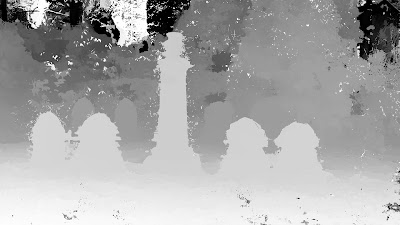Depth map obtained by DMAG8b using near plane = 0, far plane = 0, number of planes = 0, radius = 36, alpha = 0.9, truncation (color)= 30, truncation (gradient) = 10, and epsilon = 4.

I wouldn't worry too much about the wrong depths way back in the background as the actual far plane is probably deeper than the calculated far plane (from the 3D reconstruction).

Now, let's look at the depth map produced by the combo ER9b+DMAG5:

The initial pair chosen by SfM10 is the stereo pair we are gonna use in ER9b. In order to get a depth map (with DMAG5) that shows the foreground as white and the background as black, we need to figure out which one is the left image and which one is the right image. That's pretty easy to tell just by looking at the images.

The min and max disparity that ER9b outputs can be plugged in into DMAG5 as is. If they don't make sense (the range is way too large compared to reality), it definitely means that wrong matches were kept as inliers (the rectified images should be ok though). In that case, one can either try to use ER9 instead of ER9b (don't forget to negate and switch the min and max disparities since ER9 uses a different convention than ER9b for disparities) or use DF2 to manually compute the min and max disparities. In our case, ER9b gives min disparity = -8 and max disparity = 139, which looks mighty fine. Note that the initial pair chosen by SfM10 was the pair of images with index 2 and 3, which means that image 2 is the reference image. For ER9b and DMAG5, the left image is the one with index 3 and the right image the one with index 2. No big deal if you switch those up as the only thing that's gonna happen is that you're gonna get an inverted depth map where black is foreground and white is background.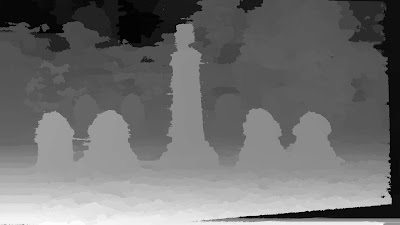Disparity map obtained by DMAG5 using radius = 36, alpha = 0.9, truncation (color)= 30, truncation (gradient) = 10, and epsilon = 4.

This depth map was obtained using the exact same parameters (in DMAG5) as the previous one (DMAG8b). The only difference really is that here (DMAG5) the left and right depth maps are computed in order to detect occlusions which are then erased and filled in using neighboring depths (inpainting).

Which depth map is best? Well, I will let you decide but one can certainly say that the depth map produced by the combo SfM10+DMAG8b is not really better than the depth map produced by the combo ER9b+DMAG5. It should also be noted that, in general, the combo SfM10+DMAG8b is much slower than the combo ER9b+DMAG5.

### Structure from Motion - Tombs and memorials (2)

Here are some 3D scene reconstructions that were obtained with Structure from Motion 10 (SfM10). The frames were extracted from short movies taken by my iphone 4 (resolution=1920x1080). To extract the frames from the videos, I used avidemux, but anything else can be used like VLC.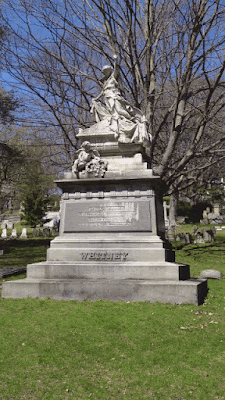Input to SfM10: 7 frames at a resolution of 1920x1080 using a focal length equal to 1920x35/36=1866.Sparse 3D scene reconstructed by SfM10 (number of trials in AC-RANSAC = 1000, max number of iterations in Bundle Adjustment = 1000, min separation angle = 1.5, and max reprojection error = 16).

Hmmm, something went terribly wrong here. Let's hike up the number of trials in AC-RANSAC and see if that's enough to solve the problem.Sparse 3D scene reconstructed by SfM10 (number of trials in AC-RANSAC = 10000, max number of iterations in Bundle Adjustment = 1000, min separation angle = 1.5, and max reprojection error = 16).

Looks like it solved the problem nicely.Input to SfM10: 7 frames at a resolution of 1920x1080 using a focal length equal to 1920x35/36=1866.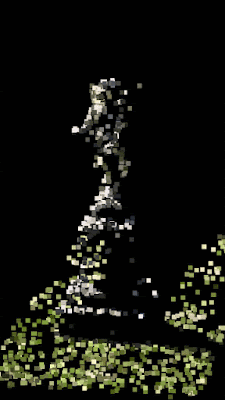Sparse 3D scene reconstructed by SfM10 (number of trials in AC-RANSAC = 1000, max number of iterations in Bundle Adjustment = 1000, min separation angle = 1.5, and max reprojection error = 16).Input to SfM10: 6 frames at a resolution of 1920x1080 using a focal length equal to 1920x35/36=1866.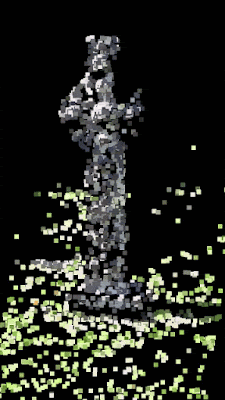Sparse 3D scene reconstructed by SfM10 (number of trials in AC-RANSAC = 1000, max number of iterations in Bundle Adjustment = 1000, min separation angle = 1.5, and max reprojection error = 16).Input to SfM10: 5 frames at a resolution of 1920x1080 using a focal length equal to 1920x35/36=1866.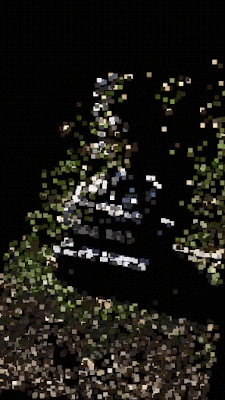Sparse 3D scene reconstructed by SfM10 (number of trials in AC-RANSAC = 1000, max number of iterations in Bundle Adjustment = 1000, min separation angle = 1.5, and max reprojection error = 16).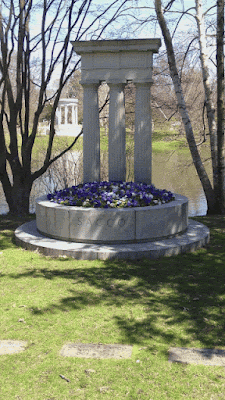Input to SfM10: 8 frames at a resolution of 1920x1080 using a focal length equal to 1920x35/36=1866.Sparse 3D scene reconstructed by SfM10 (number of trials in AC-RANSAC = 1000, max number of iterations in Bundle Adjustment = 1000, min separation angle = 1.5, and max reprojection error = 16).

Recall that the nvm file that SfM10 outputs can be used to generate a dense depth map (Multi View Stereo) with Depth Map Automatic Generator 8 or Depth Map Automatic Generator 8b (DMAG8b.

### Structure from Motion - Tombs and memorials

Here are some 3D scene reconstructions that were obtained with Structure from Motion 10 (SfM10). The frames were extracted from short movies taken by my iphone 4 (resolution=1920x1080). To extract the frames from the videos, I used avidemux, but anything else can be used (VLC for example).Input to SfM10: 5 frames at a resolution of 1920x1080 using a focal length equal to 1920x35/36=1866.Sparse 3D scene reconstructed by SfM10 (number of trials in AC-RANSAC = 1000, max number of iterations in Bundle Adjustment = 1000, min separation angle = 1.5, and max reprojection error = 16).Input to SfM10: 5 frames at a resolution of 1920x1080 using a focal length equal to 1920x35/36=1866.Sparse 3D scene reconstructed by SfM10 (number of trials in AC-RANSAC = 1000, max number of iterations in Bundle Adjustment = 1000, min separation angle = 1.5, and max reprojection error = 16).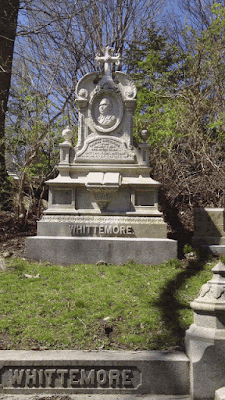Input to SfM10: 5 frames at a resolution of 1920x1080 using a focal length equal to 1920x35/36=1866.Sparse 3D scene reconstructed by SfM10 (number of trials in AC-RANSAC = 1000, max number of iterations in Bundle Adjustment = 1000, min separation angle = 0.5, and max reprojection error = 16).Input to SfM10: 8 frames at a resolution of 1920x1080 using a focal length equal to 1920x35/36=1866.Sparse 3D scene reconstructed by SfM10 (number of trials in AC-RANSAC = 1000, max number of iterations in Bundle Adjustment = 1000, min separation angle = 1.5, and max reprojection error = 16).

Recall that the nvm file that SfM10 outputs can be used to generate a dense depth map (Multi View Stereo) with Depth Map Automatic Generator 8 or Depth Map Automatic Generator 8b (DMAG8b.

## Monday, April 11, 2016

### Structure from Motion - Target aisles

Went to Target and shot a couple of videos using my iphone 4 to test Structure from Motion 10 (SfM10). The iphone 4 captures videos at a resolution of 1920x1080. To extract the frames from the videos, I used avidemux. Two mega pixels per frame is not too bad for SfM10, so I didn't downsize the frames.Input to SfM10: 4 frames at a resolution of 1920x1080 using a focal length equal to 1920x35/36=1866 (number of trials in AC-RANSAC = 1000, max number of iterations in Bundle Adjustment = 1000, and min separation angle = 0.0).Input to SfM10: 5 frames at a resolution of 1920x1080 using a focal length equal to 1920x35/36=1866 (number of trials in AC-RANSAC = 1000, max number of iterations in Bundle Adjustment = 1000, and min separation angle = 1.5).

## Saturday, April 9, 2016

### Structure from Motion 10 (SfM10)

SfM10 (Structure from Motion 10) builds a sparse 3d scene reconstruction given a set of still frames and a focal length (for each frame). Of course, it also computes the camera poses (rotation and translation) for each camera/view.

The Structure from Motion pipeline in SfM10 is as follows:
- For each camera/view, extract the features using SIFT.
- For each camera pair, compute the matches.
- For each camera pair, compute the good matches by removing outliers and keeping only the inliers. This is done using A Contrario RANSAC aka AC-RANSAC.
- Initialize the 3d scene with an adequate initial pair. The initial pair should be chosen so that the corresponding two views are not related by a homography, in other words, the baseline should be large enough (while having a good number of matches).
- Remove the spurious (low-confidence) 3d points. If the separation angle between the rays emanating from a given 3d point to the camera centers is below some threshold, the 3d point is considered unreliable and is therefore removed from the 3d reconstruction.
- Perform Bundle Adjustment on camera poses and the 3d points. The non-linear cost function is minimized with LBFGS instead of the more widely used Levenberg-Marquardt (just to be different).
- For each remaining camera:
-- Compute the camera pose using EPnP and add measurements to existing 3d points. This is called "resectioning" in Computer Vision lingo.
-- Add the 3d points seen by the camera.
-- Remove the spurious (low confidence) 3d points.